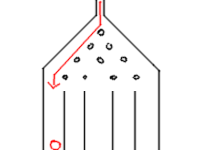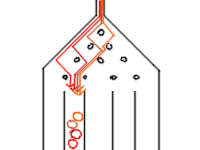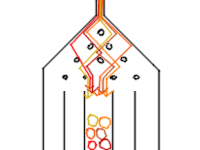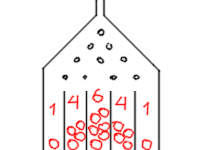# Galton board

The Galton board is a physical model of the binomial distribution which beautifully illustrates the central limit theorem

Galtonboard is also known as:

• Galtonbrett Simulation,
• quincunx
• bean machine
• or Galton

The Galtonboard is a device invented by Sir Francis Galton to demonstrate the central limit theorem, in particular that the normal distribution is a good approximate to the binomial distribution.

The ball is not the variable. The variable is whether it goes left or right. Every ball is randomly pushed left and right.

The galtonboard demonstrates that the binomial distribution is approximately a normal distribution.

Invented by Sir Francis Galton (1822-1911), the Galton board is a vertically placed rectangular board with evenly spaced pegs or nails.

## ExplicationThere's only one way for a ball to reach the first columnThere are four ways to reach the second columnThere are six ways to reach the third columnBecause the machine is symetrical, after some time it will look like a gaussian distribution

## Documentation / Reference

Recommended Pages(Probability|Statistics) - Binomial Distribution

The binomial distribution is the discrete probability distribution of the number of successes in a sequence of n independent yes/no experiments, each of which yields success with probability p. The...Statistics - (Normal|Gaussian) Distribution - Bell Curve

A normal distribution is one of underlying assumptions of a lot of statistical procedures. In nature, every outcome that depends on the sum of many independent events will approximate the Gaussian distribution...Statistics - Central limit theorem (CLT)

The Central_limit_theoremcentral limit theorem (CLT) is a probability theorem (unofficial sovereign) It establishes that when: random variables (independent) (estimate of a random process) are added...